ESDEP WG 10

COMPOSITE CONSTRUCTION

# Lecture 10.6.3: Shear Connection III

#### OBJECTIVE/SCOPE

To explain the design of shear connection in continuous composite beams, with special emphasis on hogging moment regions.

#### PREREQUISITES

Lectures 10.6: Shear Connection

#### RELATED LECTURES

Lectures 10.4: Continuous Beams

Lectures 10.5: Design for Serviceability

#### SUMMARY

The aim of the lecture is to explain simply the basis of designing full and partial shear connection in continuous composite beams, mainly dealing with the connector distribution in hogging moment regions. The greatest care must be taken regarding the validity of plastic cross-section behaviour. For critical cross-sections in Class 1, a general method is developed, illustrated by examples. For critical cross-sections in Class 2, an outline is given using a simplified method.

## 1. INTRODUCTION

This lecture describes the design of shear connection in continuous composite beams. The problem is discussed mainly with regard to a practical method for determining the connector distribution along the shear span in the hogging moment region. The use of partial shear connection in a continuous beam is considered; this is a complicated problem which must be related to the validity of plastic theory and take account of the increased risk of local flange buckling at internal supports.

### 2.1 General Aspects

The design of the shear connection in a continuous beam is more complicated than for a simply supported beam because of hogging bending effects over the internal supports. The cross-sections in these regions may become critical and have to be checked. Checking them is complicated by the decrease in bending stiffness (due to slab cracking) and the tendency for the neutral axis to move up the steel web, if slab reinforcement is used, thereby changing the classification of the section elements (according to the definitions given in Lecture 10.3). In such situations, the scope for the application of rigid-plastic analysis of beams is more restricted. There is then the need to distinguish between several procedures for connection design.

Before describing the procedures the following points should be noted:

• Even if an elastic analysis is used for the continuous beam (possibly in conjunction with redistribution of support moments), plastic design of the shear connection is not completely excluded when the end cross-sections of any critical length are at least in Class 2. This is because these cross-sections are able to develop their plastic moment resistances without risk of local buckling - see Section 3.
• In hogging bending regions, design of partial shear connection is difficult to check with regard to the required rotation capacities of the cross-sections at internal supports, even if these cross-sections are in Class 1. Therefore, to conform with Eurocode 4 , it is preferable not to allow partial interaction in hogging regions, in order to reduce the risk of local buckling of steel.
• In sagging bending regions, if the cross-sections at internal supports are in Class 2, 3 or 4, partial shear connection is sufficient because the maximum positive moment due to design loads is clearly less than the plastic moment resistance Mu. This assumes that elastic global analysis is used and that there are no special restrictions as, for example, apply in the case of bridge beams.
• Experimental work indicates that when the slab is in tension the shear connection is less stiff and the ultimate resistance is slightly reduced . This behaviour is the reason why some codes (such as the British Standards) propose, for composite beams in buildings, that the ultimate resistance of connectors PRd in hogging bending regions of continuous beams should be taken as about 20 per cent less than the value PRd used in sagging bending regions. However, Eurocode 4 does not have such a requirement.

### 2.2 Continuous Beams with Critical Cross-Sections in Class 1

The very simple example of a two-span continuous beam under concentrated loading is taken below to illustrate the procedures.

#### 2.2.1 Simple Case - Single Point Load Only

Figure 1a shows a span AC of a two-span composite beam, loaded by a single concentrated load Q, at point B. A design method is now developed for both partial and full shear connection of the beam. At failure, a complete plastic mechanism is assumed to occur (Class 1 sections), see Figure 1b, with the bending moment reaching the ultimate cross-section resistance at each plastic hinge location. If these moments are assumed to be Mu(r) and M¢u, in sagging and hogging respectively (see Figure 1c), then the ultimate load is given by the relationship:

Q = {Mu(r)L + M¢ud}/[d(L - d)]       (1)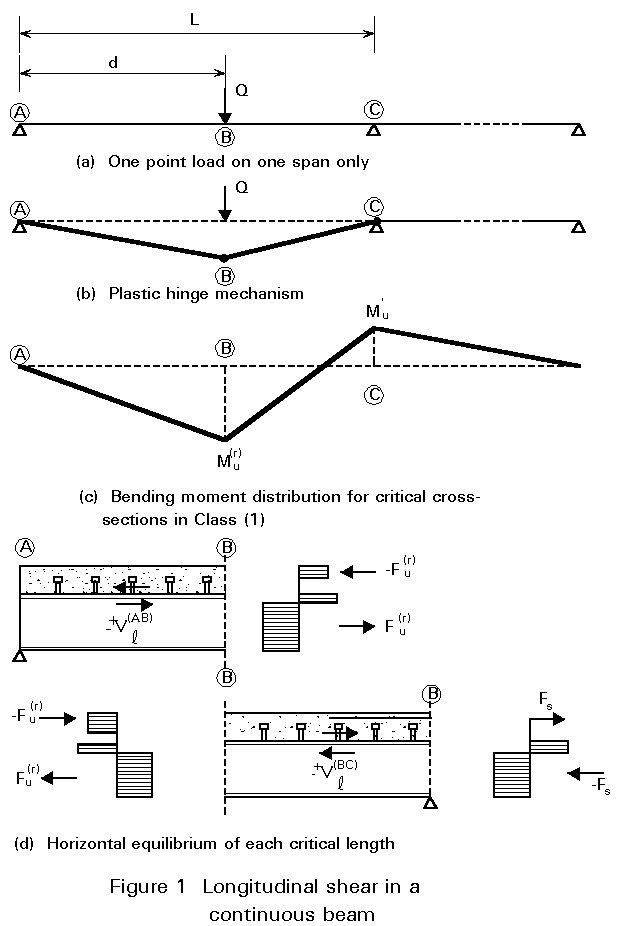Clearly, the values of Mu(r) and M¢u depend on the arrangement of the shear connection. As explained in Lecture 10.4.1, the calculation of the hogging moment of resistance M¢u is based on the following assumptions:

• tension stresses in the concrete are neglected.
• the reinforcement is yielding, so the tensile force in the reinforcement at failure is equal to:

Fs = asfsk /gs         (2)

• all fibres of the steel section are yielding either in compression or tension.

The critical length BC, where the bending moment changes sign, is considered first. The number N(BC) of ductile shear connectors uniformly distributed along this critical length can be chosen. The horizontal equilibrium condition (see Figure 1d), for the concrete slab, gives the equation:

Vl(BC) = N(BC) PRd = Fu(r) + Fs (3)

where Vl(BC) is the total longitudinal shear force in the critical length considered, and Fu(r) is the compressive force in the concrete slab in the internal critical cross-section B. Hence, the value of Fu(r) can be determined:

Fu(r) = N(BC) PRd - Fs (4)

and consequently the reduced ultimate sagging moment Mu(r) by using Equations (10) and (12) established for simply supported beams (see Lecture 10.6.2).

Considering the external critical length AB, which is only in sagging bending, the required number N(AB) of shear connectors follows directly from the Equation:

Vl(BC) = N(AB) PRd = Fu(r), (5)

hence:

N(AB) = Fu(r)/PRd = N(BC) - Fs/PRd (6)

Finally, the total number N of shear connectors uniformly distributed along the whole span AC is equal to:

N = N(AB) + N(BC) = 2 N(BC) - Fs/ PRd  (7)

In summary, using the parameter N(BC), the ultimate load Q of the beam, Equation (1), can be calculated as a function of the total number N of shear connectors, Equation (7), in the span concerned by assuming a plastic failure mechanism occurs. Obviously, the case of full shear connection corresponds to the particular value Nf(BC) such that Equation (4) leads to the maximum value of Fu(r), i.e. Vlf given by Equation (4) Lecture 10.6.2. Therefore:

Nf(BC) = (Vlf + Fs)/PRd             (8)

The corresponding values of the total number of shear connectors and the ultimate load of the beam are defined by symbols Nf and Qu.

To illustrate the above results, Figure 2 gives a graphical representation of the relation between Q and N (in non-dimensional form), namely the qualitative curve A¢B¢C¢ . For the same reasons as explained in the case of simply supported beams, the whole curve A¢B¢C¢ is not usable; as before, the minimum number of shear connectors must be limited to a function of the span L of the beam. Therefore: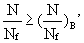(9)

where the limitation (N/Nf)B¢ may again be evaluated by means of Equations (15) and (16) given in Lecture 10.6.2. Such an evaluation is conservative because partial shear connection deals here only with the region in sagging bending, and this region is shorter than L.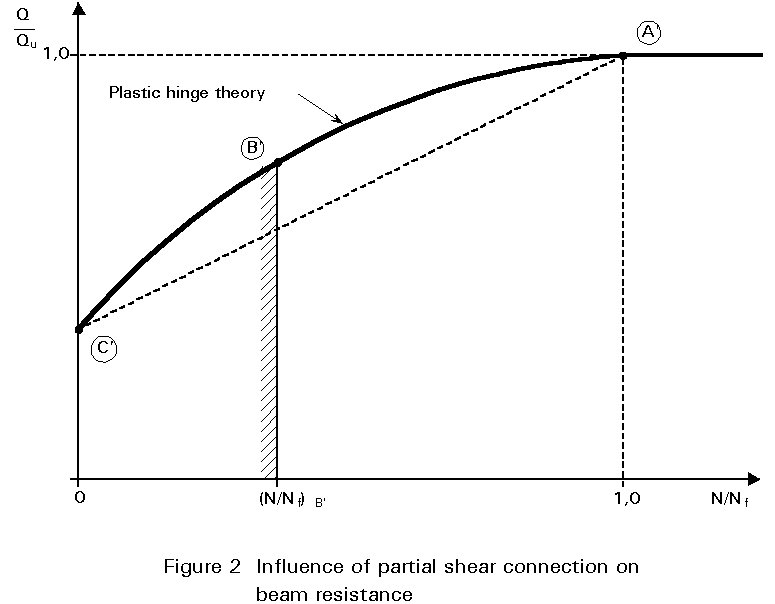The following observations are made regarding the above approach:

(i) The formulae derived are based on an assumed zero value for the compressive force possibly developed in the concrete at the intermediate support. This is a conservative assumption and an increase in design resistance may result from a more detailed analysis .

(ii) The curve A¢B¢C¢ shown in Figure 2, is always convex, like curve ABC in Fig. 4 of Lecture 10.6.2. This property results directly from Equation (1) and the negative value of the second derivative of function Mu(r)(N(AB)), and consequently function Mu(r)(N). A simplified method can be proposed for the determination of the ultimate load Q of the continuous beam with partial shear connection. This method is again based on linear interpolation between the ultimate load Qapl of the steel beam alone and the ultimate load Qu of the composite beam with full shear connection:

Q = Qapl +(Qu - Qapl) (10)

(iii) As has already been explained, Q can be calculated when the number of shear connectors and their distribution, is known. Vice versa, when Q is fixed, curve A¢B¢C¢ or line A¢C¢ allows the determination of the total number N of shear connectors and their distribution can then be determined from Equation (7), then (6).

(iv) The discussion thus far has concentrated on the particular load/span arrangement shown in Figure 1. However, it is clear that the same principles can easily be applied to other more general cases of continuous beams. For these cases, Equation (1) (used to calculate the ultimate load) will be different and the definition of the critical lengths related to the plastic mechanism at failure may be more subtle. Section 2.2.2 covers situations where several point loads and/or distributed loads are applied on any span of a continuous beam.

#### 2.2.2 General Cases

Figure 3 shows the case of two equal point loads each located at a quarter span from the supports. The cross-section at point B is the critical one and the corresponding ultimate load is given by the relationship:

Q = (4Mu(r) + M¢u)/L              (11)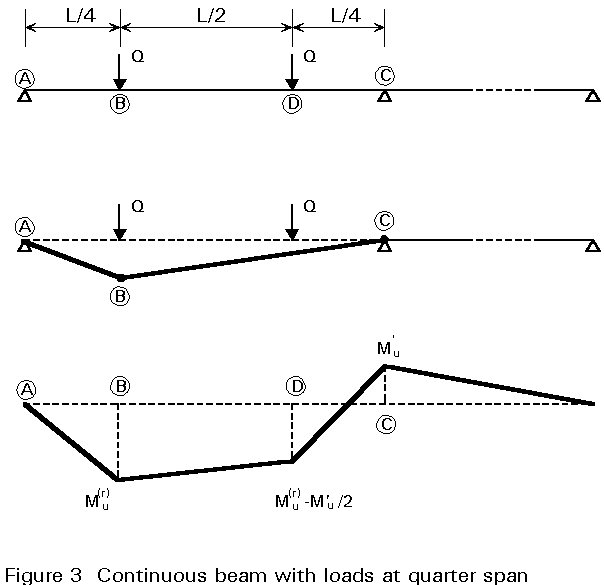However, if the ultimate value of Q is relatively large, compared to the section resistance, an intermediate cross-section must be introduced at point D, resulting in the connectors not being spaced uniformly along the internal critical length BC.

Figure 4 shows a symmetrical two-span beam with a uniformly distributed load "q". The shear connection design requires that the location of the critical cross-section B should be calculated from consideration of the plastic hinge mechanism. Therefore:

d = L(Mu(r)/M¢u){Ö[1+ (M¢u/Mu(r))]-1}     (12)

and the corresponding value of the ultimate load is:

q = 2[Mu(r)L + M¢ud]/[d(L-d)L]              (13)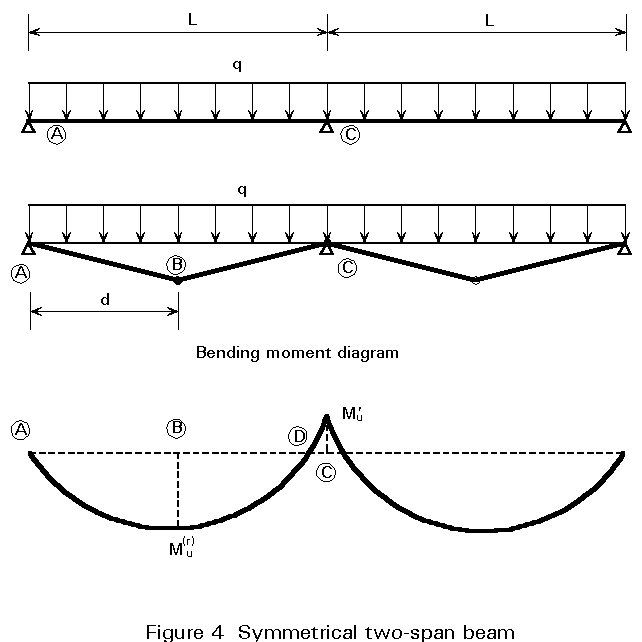In contrast to Figures 1 and 3, here the location of the critical cross-section B is slightly affected by the choice of the number N(BC) of shear connectors; this makes the determination of the curve (q/qu, N/Nf), similar to curve A¢B¢C¢ in Figure 2, more difficult. The interpolation method, however, is still valid, symbol Q being replaced by symbol q in Equation (10).

Finally, Figure 5 shows a more complicated case: an unsymmetrical two-span beam on which a uniformly distributed load q is applied together with a heavy point load Q on the longer span only. In this case, the position of critical cross-sections B and B¢, in sagging moment, should be found (see d and d¢ in the Figure), and the critical length AB should be divided into two sub-lengths by introducing the intermediate cross-section D for checking the adequacy of the shear connection. The internal critical length BC can be designed in accordance with the general method or the simplified one. For the internal critical length CB¢, which is not part of the plastic hinge mechanism, its shear connection can be designed by interpreting the external bending moment MB¢ at failure as equal to a reduced plastic resistance moment in the cross-section B¢. It should be noted that the same interpretation is used for intermediate cross-sections.## 3. CASE OF CONTINUOUS BEAMS WITH CRITICAL CROSS-SECTIONS IN CLASS 2 AT INTERNAL SUPPORTS

Class 2 cross-sections are discussed here together with cross-sections with Class 3 webs which can be replaced by an effective web in Class 2 using area reduction. Referring to the simple example of Figure 1, the ultimate design load for the beam cannot be calculated using plastic hinge analysis. Only an elastic global analysis with limited redistribution of moments can be used for the beam, which leads to an ultimate load Qd less than the corresponding value Qu for a Class 1 beam with full shear connection. Since MB < Mu in the sagging moment region, as shown in Figure 6, it is clear that partial shear connection will occur even when the beam is used to its full flexural resistance. Attention must be paid here to the fact that the convex curve A¢B¢C¢, in Figure 2, cannot be used to calculate the total number N of shear connectors in span AC because, the plastic hinge mechanism cannot occur at the Class 2 cross-section B. In practice, however, a line similar to A¢C¢ can be used for this problem, as it gives safe results. Therefore, in the absence of a more rigorous calculation, the degree of connection N/Nf may be calculated from the linear relationship:

N/Nf  = (Qd - Qapl)/(Qu - Qapl) (14)

It should be noted that Qu and Qapl are calculated now using elastic global analysis.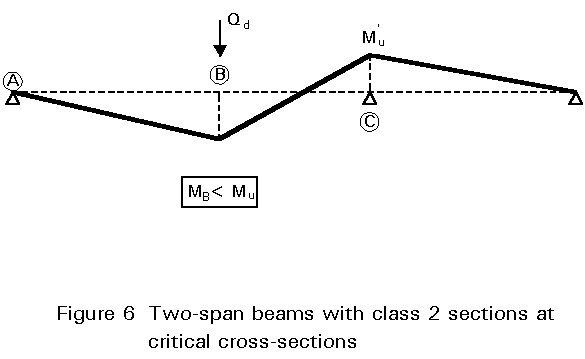## 4. CONCLUDING SUMMARY

• Shear connection design, for hogging moment regions of continuous composite beams, is a more complex problem than for the simply supported case and requires special analysis.
• When ductile shear connectors are used a plastic design method can be developed for use in the hogging moment regions, which depends on whether a Class 1 or 2 beam is being used. To avoid complex analysis a simple linear method can be used for design.

## 5. REFERENCES

 Eurocode 4: "Design of Composite Steel and Concrete Structures": ENV1994-1-1: Part 1.1: General rules and rules for buildings, CEN (in press).

 Johnson, R.P., "Composite Structures of Steel and Concrete - Vol. 1." Crosby Lockwood, 1975.

 Stark, J.W.B., "Composite Steel and Concrete Beams with Partial Shear Connection", Heron, Vol. 34, No. 4, 1989.